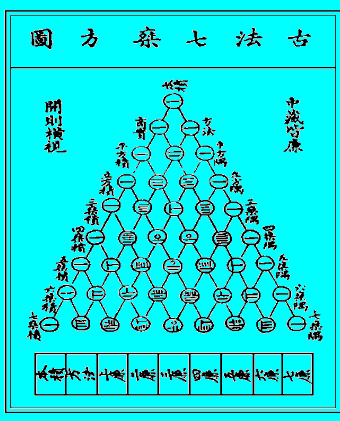# Shoeless Trivia: Math

In a hurry today, and math is a low research area for me, so….

1. Name either of the two mathematicians (one German, one English) generally credited with discovering calculus.
2. The mathematical structure shown below is named for what 17th century French mathematician, even though it was known centuries earlier in China, India, and elsewhere?3. What is the sum (in  degrees) of the exterior angles of a regular 17-gon?
4. “The sum of the square roots of any two sides of an isosceles triangle is equal to the square root of the remaining side” is an (incorrect) mathematical pronouncement made by a character in what film shortly after being awarded an advanced degree?
5. Now for the most wickedly difficult question of them all. Complete the following statement: The red curve shown below is known as the BLANK of Agnesi, named for Maria Agnesi and described in her book Instituzioni analitiche ad uso della gioventù italiana.